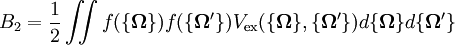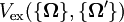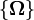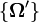2022: SklogWiki celebrates 15 years on-line

# Osmotic virial coefficientsThis article is a 'stub' page, it has no, or next to no, content. It is here at the moment to help form part of the structure of SklogWiki. If you add sufficient material to this article then please remove the {{Stub-general}} template from this page.$B_2 = \frac{1}{2} \iint f( \{ {\mathbf \Omega} \}) f( \{ {\mathbf \Omega}' \}) V_{\rm ex} ( \{ {\mathbf \Omega} \},\{ {\mathbf \Omega}' \}) d \{ {\mathbf \Omega} \} d \{ {\mathbf \Omega}' \}$
where$V_{\rm ex} ( \{ {\mathbf \Omega} \},\{ {\mathbf \Omega}' \})$ is the excluded volume for two polymers with conformations$\{ {\mathbf \Omega} \}$ and$\{ {\mathbf \Omega}'\}$. The curly braces denotes an ensemble of molecular configurations for a single polymer.